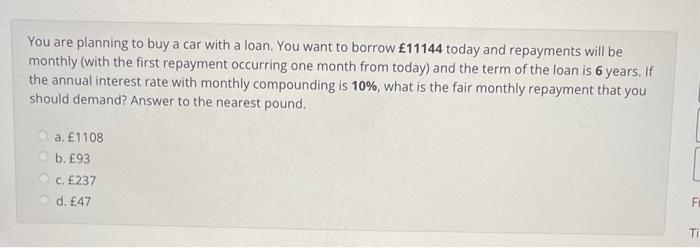# Question You are planning to buy a car with a loan. You want to borrow $$£ 11144$$ today and repayments will be monthly (with the first repayment occurring one month from today) and the term of the loan is 6 years. If the annual interest rate with monthly compounding is $$10 \%$$, what is the fair monthly repayment that you should demand? Answer to the nearest pound. a. $$£ 1108$$ b. $$£ 93$$ c. $$£ 237$$ d. $$£ 47$$Transcribed Image Text: You are planning to buy a car with a loan. You want to borrow $$£ 11144$$ today and repayments will be monthly (with the first repayment occurring one month from today) and the term of the loan is 6 years. If the annual interest rate with monthly compounding is $$10 \%$$, what is the fair monthly repayment that you should demand? Answer to the nearest pound. a. $$£ 1108$$ b. $$£ 93$$ c. $$£ 237$$ d. $$£ 47$$
Transcribed Image Text: You are planning to buy a car with a loan. You want to borrow $$£ 11144$$ today and repayments will be monthly (with the first repayment occurring one month from today) and the term of the loan is 6 years. If the annual interest rate with monthly compounding is $$10 \%$$, what is the fair monthly repayment that you should demand? Answer to the nearest pound. a. $$£ 1108$$ b. $$£ 93$$ c. $$£ 237$$ d. $$£ 47$$&#12304;General guidance&#12305;The answer provided below has been developed in a clear step by step manner.Step1/4Given Data: -Requirement: -Explanation:Please note that this is an ordinary annuity payment as the first payment occurs one month from today.Step2/4Calculate the Effective Annual Rate: -Given an Annual Interest Rate with Monthly Compounding first determine the Effective Annual Rate as follows: -\begin{align*} \mathrm{{E}{A}{R}} &= \mathrm{{\left({1}+\frac{{R}}{{T}}\right)}^{{{T}}}-{1}} \end{align*}Where $$\mathrm{{E}{A}{R}}$$ is the Effective Annual Rate.Where $$\mathrm{{R}}$$ is the Annual Interest Rate with Monthly Compounding.Where $$\mathrm{{T}}$$ is the number of months in a year.\begin{align*} \mathrm{EAR} & = \mathrm{{\left({\left({1}+\frac{{0.1}}{{12}}\right)}^{{12}}-{1}\right)}} \\& = \mathrm{{0.1}} \end{align*}Explanation:First find the Effective Annual Rate given an Annual Interest Rate with Monthly Compounding as this will be used in the following steps.Step3/4Calculate the Number of Periods: -Given the number of years are 6 and the number of months per year are 12 the number of periods are calculated as follows: -\begin{align*} \mathrm{N\space } & = \mathrm{T\space *\space M} \\& = \mathrm{{6}\times{12}} \\& = \mathrm{{72}} \end{align*}Where $$\mathrm{{N}}$$is the Number of Periods.Where \( ... See the full answer